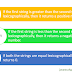The Java String Class provides many ways to compare the content of the two Strings. One way to compare the content of the two strings by using the equals() method. We have learned the equals() method in our last tutorial. In this tutorial, we will learn how to compare two strings lexicographically in Java.

## Java String compareTo() Method

Java String compareTo() method is used to compare the two strings lexicographically in Java. The comparison is based on the Unicode value of each character in the string. The String compareTo() method returns the positive number, negative number or 0.

If the first string is greater than the second string lexicographically, then it returns a positive number. If the first string is smaller than the second string lexicographically, then it returns a negative number. If both the strings are equal lexicographically, then it returns 0.

## Java program to compare two strings lexicographically

``````class Lexicographically{
public static void main(String args[]){
String str1 = "Hello";
String str2 = "Hi";
String str3 = "Ram";
String str4 = "Javastudypoint";
String str5 = "Tutorial";
String str6 = "Tutorial";

//-4 because "e" is 4 times less than "i".
System.out.println(str1.compareTo(str2));

//2 because "T" is 2 times greater than "R".
System.out.println(str5.compareTo(str3));

//-2 because "H" is 2 times less than "J".
System.out.println(str1.compareTo(str4));

//-12 because "H" is 12 times less than "T".
System.out.println(str1.compareTo(str5));

//0 because both the strings are equal.
System.out.println(str5.compareTo(str6));
}
}
``````

Output:
``````
-4
2
-2
-12
0
``````
How to Compare two Strings lexicographically in Java?Reviewed by Prashant Srivastava on January 08, 2020 Rating: 5